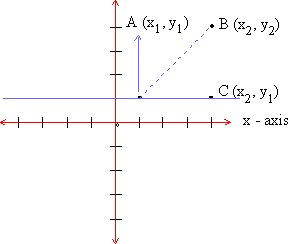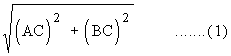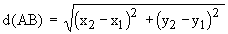Home MonkeyNotes Printable Notes Digital Library Study Guides Message Boards Study Smart Parents Tips College Planning Test Prep Fun Zone Help / FAQ How to Cite New Title Request

 9.4 Distances and Distance Formula With the help of x and y axis we saw how the position of a point in the co-ordinate plane was determined. We shall now extend this theory to calculate the distances between any two points in the co-ordinate plane. Let A (x, y) and B (x2, y2) be two points in the co-orrdinate plane as shown below :Figure 9.4 In order to find the distance between points A and B we go through following steps and use the distance formula. Step 1 Draw a line parallel to x-axis through the point A and            draw a line parallel to y-axis through point B such that           they intersect at point C. Step 2 We now have a right triangle with seg AB as the            hypotenuse. Therefore, by Pythagorean theorem,             (AB)2 = (AC)2 + (BC)2             \ AB =Step 3 The co-ordinates of point C can be determined            as (x2, y1). Step 4 The distance between A and C is,             AC = (x2, x1)             \ (AC)2 = (x2, x1)2        and             The distance between B & C is             BC = (y2, y1)             \ (BC)2 = (y2, y1)2 Step 5 Substituting the values of (AC)2 and (BC)2 in             eg (1) we getThis is the distance formula. Index 9.1 Points And Co-ordinates 9. 2 Co-ordinates and Axes 9. 3 Quadrants 9. 4 Distances And Distances Formula 9. 5 Mid Point Formula 9. 6 Slope Of A Line 9. 7 Equation Of A Line Chapter 1
 Search: All Products Books Popular Music Classical Music Video DVD Toys & Games Electronics Software Tools & Hardware Outdoor Living Kitchen & Housewares Camera & Photo Cell Phones Keywords: